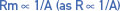Want create site? Find Free WordPress Themes and plugins.

Some materials require high magnetizing forces to align their atomic dipoles in the same direction, while others are readily magnetized by small forces. All materials offer some opposition to being magnetized and the term used to describe this opposition is ‘magnetic reluctance’.

## Magnetic Reluctance Formula

Reluctance is comparable with resistance in an electric circuit and, like resistance, depends on a number of factors:

 1 Length of a magnetic circuit. Reluctance varies directly with the mean length of a magnetic circuit and is similar in this respect to electrical resistance:2 Cross-sectional area of a magnetic circuit. Reluctance varies inversely with the cross-sectional area of a magnetic circuit:3 Permeability of the circuit material. The term permeability is used as a measure of the ease with which materials may be re-magnetized. Reluctance, on the other hand, is a measure of the opposition to flux. Permeability is analogous to the resistivity of an electric circuit:where: Rm = reluctance in ampere-turns per weber l = length of circuit in meters A = cross-sectional area in square meters µo = permeability of free space µr = relative permeability

## Magnetic Permeability Example

The total mean length of the path of an iron core is 200 mm. The core is rectangular in cross-section with dimensions 15 mm × 10 mm. If the core has a relative permeability of 830 at the designed flux density, calculate the reluctance of the core. (Remember to convert to base units.)Did you find apk for android? You can find new Free Android Games and apps.